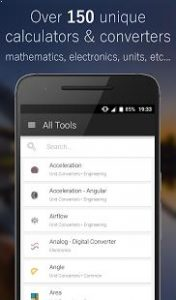# CalcKit – Over 150 unique calculators and unit converters

## [App] CalcKit – All-in-One CalculatorMeet CalcKit, the most powerful All-in-One Calculator Pack for Android.

Featuring over 150 unique calculators and unit converters, packed in with a highly customizable scientific calculator and even letting you build your own calculators and converters, CalcKit is the All-in-One Calculator you’ve been dreaming about.

These features, combined with an intuitive and eye-catching design, make CalcKit stand out from its competitors.

In addition to everyday use, CalcKit is suitable for a large variety of professions. If you’re a scholar, student, teacher, builder, handyman or engineer and you need an All-in-One Calculator App for your device, give CalcKit a try.

CalcKit Features
Over 150 Calculators and Unit converters
Highly customizable Scientific & RPN Calculator
Floating Calculator Widget
Fully editable Calculator history
Light & Dark Calculator Themes
Currency converter with 160 currencies, available offline
Supports math expressions as input
Instant results
Unique Custom Tools Creator with up to 25 variables
Integrated Search

Languages:
English, Chinese, French, German, Greek, Italian, Macedonian, Russian, Serbian, Spanish, Turkish

List of calculators and converters

Geometry 2D
Triangle, Right Triangle, Square, Rectangle, Parallelogram, Rhombus, Trapezoid, Isosceles Trapezoid, Hexagon, Polygon, Ellipse, Circle, Segment and Sector of Circle

Geometry 3D
Cube, Cuboid, Prism, Tetrahedron, Octahedron, Pyramid, Pyramidal Frustum, Cone, Conical Frustum, Cylinder, Torus, Sphere, Spherical Cap, Sector, Segment and Wedge

Equations
2×2 and 3×3 Systems

Analytical Geometry
Distance between 2 points
Area of Triangle
Equation of Circle and Sphere

Algebra
Prime Number Check
Proportion Calculator
Decimal to Fraction
Fraction Simplifier
Prime Factorization
GCF / LCM

Matrix Calculator
Determinant, Inverse, Transpose
A × B, A + B, A – B

Electronics
Resistor and Inductor Color Codes
LED Resistor Calculator
Series / Parallel Components
Ohms Law
Power Triangle
Y – Δ Transformation
Voltage Divider
Voltage Regulator
Operational Amplifier
NE555 Timer
Filters
Reactance
Wire Resistivity
Transformer Ratio
Battery Life Calculator
Analog – Digital Converter
Frequency Calculator

Finance
Currency Converter
VAT / Tax Calculator
Tip Calculator

Common Converters
Numbers
Roman Numerals
Prefixes
Angle
Area
Cooking
Data Storage
Fuel Consumption
Mass / Weight
Length / Distance
Temperature
Time
Speed
Pressure
Power
Volume
Force
Energy / Work

Electricity Converters
Charge
Current
Conductance
Conductivity
Field Strength
Potential
Resistance
Resistivity
Capacitance
Inductance
Charge Density (Linear / Surface / Volume)
Current Density (Linear / Surface)

Engineering Converters
Acceleration
Angular Acceleration and Velocity
Airflow
Density
Moment of Inertia
Specific Volume
Torque

Fluids Converters
Concentration (Molar / Solution)
Flow
Flow (Mass / Molar)
Mass Flux Density
Permeability
Surface Tension
Viscosity (Dynamic / Kinematic)

Heat Converters
Fuel Efficiency (Mass / Volume)
Heat Density, Flux Density, Transfer Coefficient
Specific Heat Capacity
Temperature Interval
Thermal Conductivity, Expansion and Resistance

Light Converters
Illumination
Luminance
Luminous Intensity

Magnetism Converters
Field Strength
Flux and Flux Density
Magnetomotive Force

Absorbed Dose
Activity
Exposure

Other Converters
Frequency
Image Resolution
Pace
Pixel Density
Sound
Typography
Volume (Dry / Lumber)

CalcKit user reviews :

I forgot so much basic math and calculations of %, areas, etc, of my high school and college, but I knew it was always floating somewhere in my brain. This comprehensive app found it, and allowed me to remember higher math skills from 30 years ago. So as long as the laws of physics are still around, then this is a great app for helping my granddaughter with homework or just figuring out everyday home projects. Plus, I have not even explored the multitude of subjects this toolbox offers. I just spent hours playing with its different categories. Don’t know why I posted this, but it’s well deserved.

All the lil things you need

Absolutely amazing. I’d rate this 10 stars if I could

Contact developer :

Email

Video :

Subscribe to our mailing list and get new android apps / games and updates to your email inbox.

Thank you for subscribing.

Something went wrong.